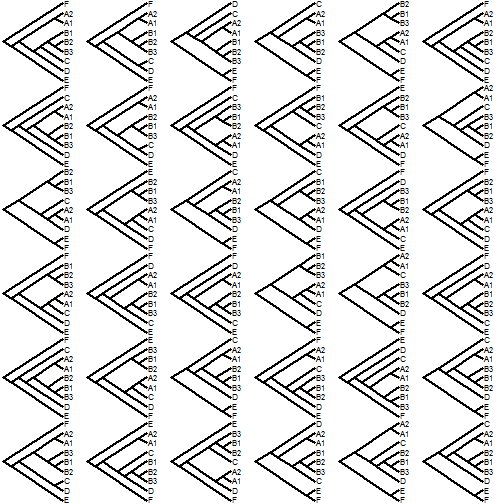## Friday, June 30, 2017

### Plotting a legend outside the plot area using `add.color.bar` (& in general)

Recently I received the following question:

“I am using the add.color.bar function to add a colorbar to an existing plot.
Do you know if it is possible to move the added colorbar to the outside of the plot domain? Do you think I would need to modify code in the actual function?”

Evidently, this can be done using `par(xpd=TRUE)`. For instance:

``````library(phytools)
tree
``````
``````##
## Phylogenetic tree with 26 tips and 25 internal nodes.
##
## Tip labels:
##  A, B, C, D, E, F, ...
##
## Rooted; includes branch lengths.
``````
``````x
``````
``````##           A           B           C           D           E           F
##  3.09148522  1.54096027  2.91508379  2.00469068  1.15813551  0.29543463
##           G           H           I           J           K           L
##  0.27951056  0.60805259 -2.52405841 -3.50891295 -3.34674083 -0.97428407
##           M           N           O           P           Q           R
## -1.25336677 -1.40812351 -0.51371266 -2.92582374 -2.24608676 -0.53203275
##           S           T           U           V           W           X
## -0.59487179  0.30811419 -0.08364322  0.16895725  0.70189973 -3.64724619
##           Y           Z
## -3.65517946 -4.30271550
``````
``````obj<-contMap(tree,x,plot=FALSE)
plot(obj,legend=FALSE,mar=c(5.1,2.1,2.1,2.1))
par(xpd=TRUE)
digits=3,prompt=FALSE,x=0,y=-3)
``````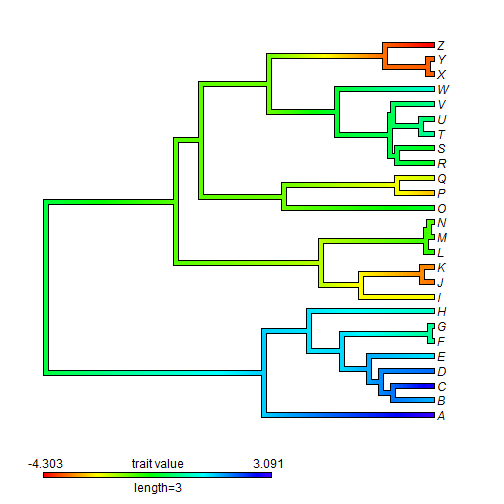We can easily see that this is outside of our plotting domain by adding axis labels to the plot as follows:

``````plot(obj,legend=FALSE,mar=c(6.1,2.1,2.1,2.1))
par(xpd=TRUE)
digits=3,prompt=FALSE,x=0,y=-4)
axis(1)
``````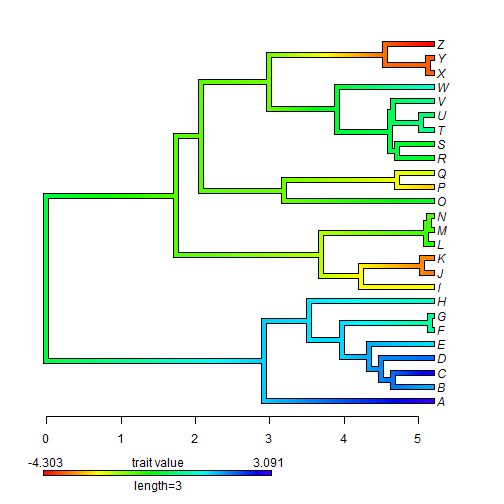You get the general idea.

### Evolution 2017 meeting slides

At the recent 'Evolution' meeting in Portland Oregon (the joint meeting of ASN, SSB, and SSE), I presented a talk entitled:

Some additional new plotting methods for phylogenies & comparative data.

The intention was to basically give a rapid-fire survey of the majority (but not all) of the phylogenetic plotting methods available in phytools, along with some comment about the purpose of different methods.

This I did; however, unfortunately, some colors were burnt out on the slide projector, so for those wondering what the plots were supposed to look like, and for those unable to attend at all, I have now posted the PDF online. The direct link is here, but the full presentation is also embedded below. Enjoy!

## Friday, June 23, 2017

### Fix to `read.newick` for cases in which some but not all nodes are labeled

It was recently reported that there can be a mismatch between the number of nodes & the length of the node label vector in an object class `"phylo"` produced by the function `read.newick` in phytools. This appears to be a bug that is produced when some nodes have labels, but the node with the largest-valued index does not have a label. I just pushed a fix to GitHub & it appears to work. Here's a demo using the case submitted on R-sig-phylo:

``````library(phytools)
``````
``````## Loading required package: ape
``````
``````## Loading required package: maps
``````
``````packageVersion("phytools")
``````
``````##  '0.6.17'
``````
``````tree<-read.newick(text='((((((a))A),(((b),(b1)))B)))C;')
tree\$edge.length<-rep(1,nrow(tree\$edge))
plotTree.singletons(tree)
nodelabels(tree\$node.label)
``````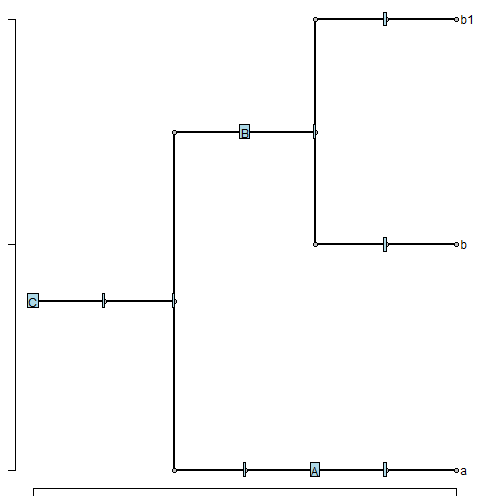That's it.

## Wednesday, June 21, 2017

### Generating a set of random resolutions of all the nodes in a tree with multifurcations

Today, an R-sig-phylo list member asked:

“I am using the ape package to randomly resolve polytomies using 'multi2di' and wondering if there is a way to use this function to get a single output tree file that contains multiple different randomly resolved trees using some number of resamplings?”

This can be done using the phytools function `resolveNode`, which returns all possible resolutions of a given node. We just iterate over all the nodes of the tree pick a random resolution each time.

Here's a function to do that:

``````resolveRandom<-function(tree){
while(!is.binary(tree)){
nodes<-1:tree\$Nnode+Ntip(tree)
Nchildren<-function(node,tree) length(Children(tree,node))
nchilds<-sapply(nodes,Nchildren,tree=tree)
node<-nodes[which(nchilds>2)]
tree<-sample(resolveNode(tree,node),1)[]
}
tree
}
``````

Here's a quick demo:

``````library(phytools)
library(phangorn)
``````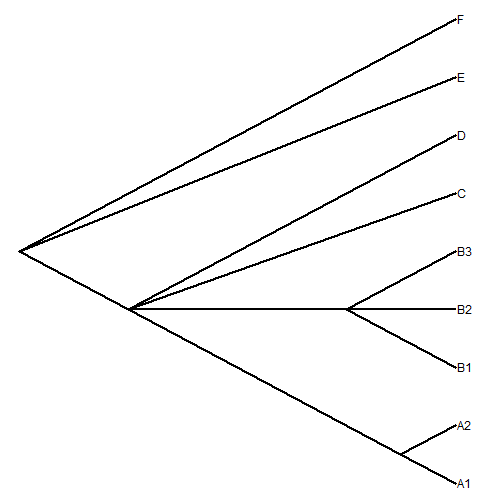``````## now some random resolutions
``````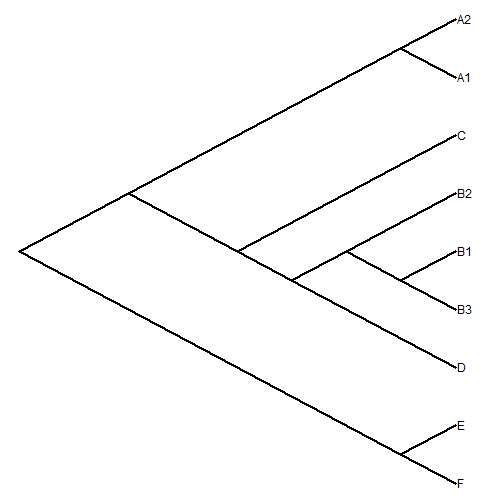``````plotTree(resolveRandom(tree),type="cladogram",nodes="centered")
``````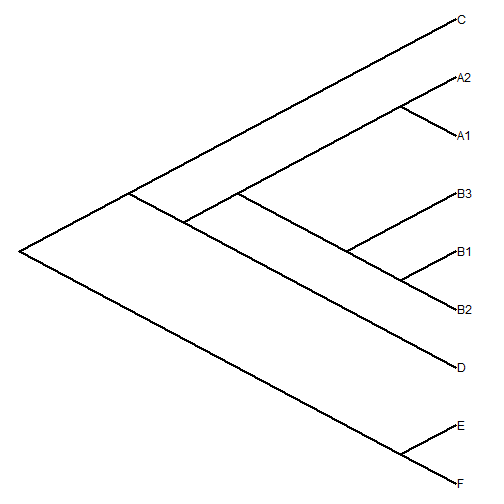``````plotTree(resolveRandom(tree),type="cladogram",nodes="centered")
``````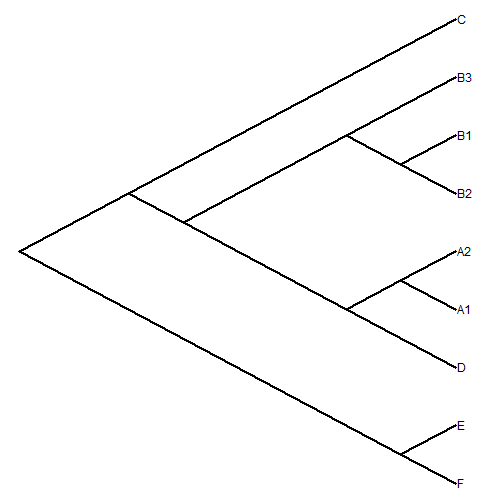``````plotTree(resolveRandom(tree),type="cladogram",nodes="centered")
``````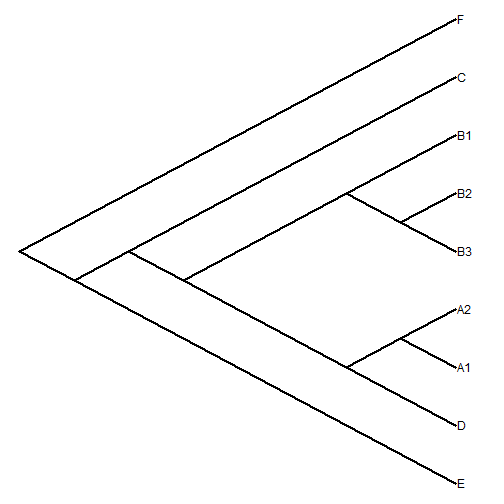We can also generate a set of resolutions using `replicate`:

``````trees<-replicate(36,resolveRandom(tree),simplify=FALSE)
class(trees)<-"multiPhylo"
par(mfrow=c(6,6))